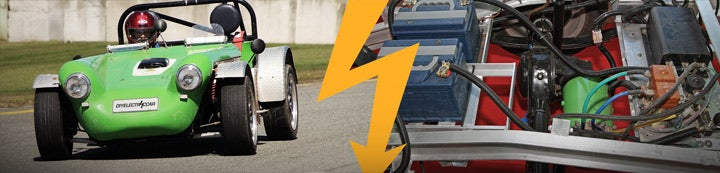1 - 5 of 5 Posts

Mufaris

·
Registered
Joined
·
1 Posts
Discussion Starter · ·
I am planning to convert a Maruthi Suzuki Esteem into pure electric car run by Switched Reluctance Motor drive .
I contacted the motor suppliers and they asked for the details such as the torque of motor required , rpm , voltage , amps .
Well from googling , i found a motor of 10 hp and 72 volt continues would be a good choice . But how to calculate it inorder to get the best performance .

The nominal speed of the car is choosen to be around 50Kmph.
Can u please tell me how to calculate the motor specifications?

Here is the car specifications

http://www.carwale.com/marutisuzuki-cars/esteem/vxibsiii20052008-specifications-261.html

kennybobby

·
Registered
Joined
·
1,499 Posts
Here is how i like to make these sort of calculatus:

1. Pick your target tires and wheels for this conversion and look up the coefficient of rolling resistance for those tires (e.g. Crr=0.010). Then calculate the circumference of the tires (pi*diameter), and determine the number of revolutions of the tire per mile. e.g for 205/65-15 tires, d=25.5 inches, C=6.673 ft/rev = 791 revs/mile.

2. Pick your desired or maximum expected speed, then calculate the wheel rpm for that speed. e.g. 50mph/60=0.833mile/minute*791rev/mile= 659 wheel rpm at 50 mph.

3. Pick a target weight, coefficient of drag and frontal area, or use the actual weight of the vehicle, Cd, and full-frontal-nudity area. Then determine the power required to operate at your maximum speed using a vehicle calculatus such as found at the economy modder site: http://ecomodder.com/forum/tool-aero-rolling-resistance.php e.g. at 50mph for W=3000 lbs (m=W/g), Crr .01, Cd .36, A 24sf, requires 11.36hp, 7.36hp aero, 4hp rr.

4. Pick desired acceleration time for 0-50mph and calculate the power needed for accel. e.g. for t=20sec the accel power is 0.5mv^2/t =12525 lb-ft/sec = 22.8 hp.

5. Sum the continuous power with accel to get peak power required, 11 +23 = 34 hp peak.

6. Calculate the wheel torque: cont torque (ft-lbs) = 11.36hp*5252/659; peak torq = 34*5252/659.

7. Pick or choose the gearbox and/or differential ratios. Divide the torques in step 6 by the gear ratio to get the required motor torques. Multiply 659 times the gear ratio to get the required motor speed.

8. You will want to add some margin on these numbers to account for losses in the gearbox, sag in the battery pack voltage, etc. The real world requirements will be slightly higher than these ideal calculations, but this will get you into the ball park of what to expect

PStechPaul

·
Registered
Joined
·
3,141 Posts
You may also try my http://enginuitysystems.com/EVCalculator.htm which also includes acceleration and slope as well as wheel diameter, torque, and ratios.

Do you have details on the switched reluctance motor and controller? I have not seen them except little ones for washing machines and very large industrial units made by Siemens.

RonMc

·
Registered
Joined
·
1 Posts
KennyBobby,

Can you please explain item 4 power to accel. I tried to follow your formula but was not able to get 12525 lb-ft/sec. use m = 3000 lb, v = 73.33 ft/sec and t = 20 sec.

Thanks

kennybobby

·
Registered
Joined
·
1,499 Posts
... to get 12525 lb-ft/sec. use m = 3000 lb, v = 73.33 ft/sec and t = 20 sec.
for W=3000 lbs, m = W/g = 93.2 lbs-sec^2/ft. where g = 32.2 ft/sec^2

1 - 5 of 5 Posts